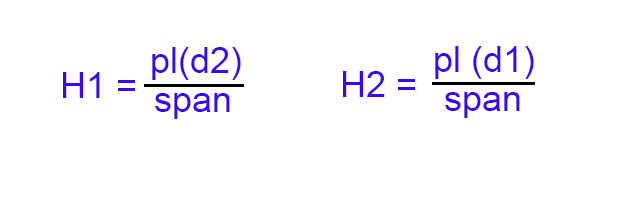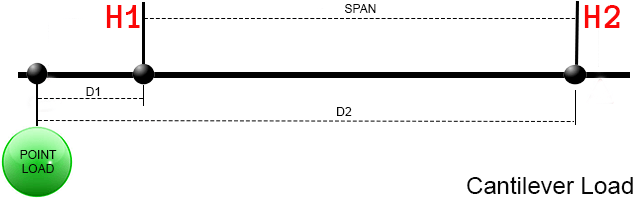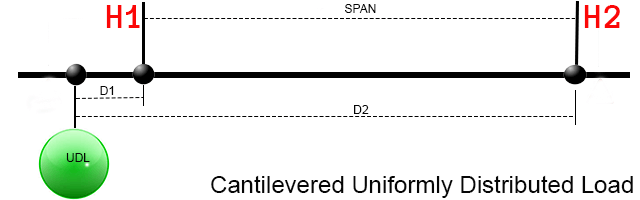﻿ LIGHTING TRUSS CALCULATIONS - STAGECRAFT PRODUCTION SERVICE### —How to hang a lighting truss—

Normally weight is computed in pounds or kilograms, lenths are coumputed in feet or meters. Always use the same unit of measure for all calculations.

As always, before suspending loads overhead consult a qualified engineer or rigger.
A speaker cabinet is an example of a single point load. In this case, the total weight of the cabinet, it's hardware and cabling is figured at 100%.An empty truss to be hung on two points is an example of an uniformly distributed load when all of the weight is between the two points. Here the weight is evenly divided between the two points.A uniformly distributed load on three points. When a third point is added, the center point carries over half of the load.The general formula for computing point loads where H1 = computed load on left point, H2 = computed load on right point, pl = the weight of the point load, d1 = distance from pl to H1, and d2 = distance from pl to H2.The following diagrams illustrate how the formula applies to various load configurations:
Point loads between two hang points. As the point load approaches a hanging point, the load on that point increases while the load on the other point decreases proportionately. The computed H1 load is added to the near point and the computed H2 load is added to the far point.Cantilever point loads. When point loads are cantilevered, the net effect results in increased load on the near point and reduced load on the far point. The computed H1 load is added to the near point and the computed H2 load is subtracted from the far point. However, where d1 is greater than span additional phantom load appears on the near point and should generally be avoided.Uniformly distributed cantilever loads. When the cantilevered point load is uniformly distributed (say for example drapery), the calculation is the same as cantilever point load however, you treat the total UDL as centered on a single point in the mid point of the cantilever. The computed H1 load is added to the near point and the computed H2 load is subtracted from the far point. However, where d1 is greater than span additional phantom load appears on the near point and should generally be avoided.